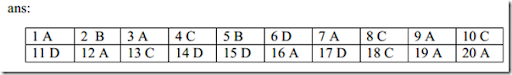Saturday, 2 August 2014

1. A number is as much greater than 36 as is less than 86. Find the number.
a)61   b) 72   c) 78   d) 87

2.Find a number such that when 15 is subtracted from 7 times the number, the  Result is 10 more than twice the number.
a)6   b) 5   c) 9   d) 7.

3. The sum of a rational number and its reciprocal is 13/6. Find the number.
a)2/3 or 3/4.  b) 2/3 or 5/2.   c) 2/5 or 3/2.  d) None

4. The sum of two numbers is 184. If one-third of the one exceeds one-seventh  of the other by 8, find the smaller number.
a)65   b) 27   c) 72   d) 89

5.  The  difference  of  two  numbers  is  11  and  one-fifth  of  their  sum  is  9.  Find  the  numbers.
a)30 and 17.  b) 28 and 17.  c) 28 and 15..  d) 28 and27.

6. . If the sum of two numbers is 42 and their product is 437, then find the  absolute difference between the numbers.
a)3  .  b) 6   c) 5.   d) 4

7. The sum of two numbers is 16 and the sum of their squares is 113. Find the  numbers.
a)7 and 8.  b) 8 and 8.  c) 9 and 8..  d) 6 and 8.

8. The average of four consecutive even numbers is 27. Find the largest of these  numbers.
(a) 24 (b) 40 (c) 30 (d) 20

9. The sum of the squares of three consecutive odd numbers is 2531.Find the  numbers.
a)27, 29 and 31  b) 21, 29 and 31  c) 27, 28 and 31.  d) 27, 29 and 35

10. Of two numbers, 4 times the smaller one is less then 3 times the 1arger one by 5.  If the sum of the numbers is larger than 6 times their difference by 6, find the two
numbers.
a)59 and 47.   b)57 and 43.   c) 59 and 43.   d) 59 and 63.

11. The ratio between a two-digit number and the sum of the digits of that  number is 4 : 1.If the digit in the unit's place is3 more than the digit in the ten’s
place, what is the number?
a)29   b) 33   c) 25.   d) 36

12. . A number consists of two digits. The sum of the digits is 9. If 63 is subtracted  from the number, its digits are interchanged. Find the number.
a)81  b) 69  c) 51.   d) 71

13. A fraction becomes 2/3 when 1 is added to both, its numerator and denominator.  And  ,it  becomes  1/2  when  1  is  subtracted  from  both  the  numerator  and
denominator. Find the fraction.
a)9 / 5  b) 8 / 5  c). 3 / 5   d) 7 / 5

14.. 50 is divided into two parts such that the sum of their reciprocals is 1/ 12.Find  the two parts.
a)50 and 20.  b) 60 and 20.  c) 40 and 20.   d) 30 and20.

15. If three numbers are added in pairs, the sums equal 10, 19 and 21. Find the  numbers
a)5, 4 and 15.  b) 6, 6and 15. c) 4, 4 and 15.  d) 6, 4 and 15.

16. What value will come in place of question mark in the following equation?
(i) 0.006 +? = 0.6
a)0.01  b) 0.02.  c) 0.03  d) 0.04

17. If (1 / 3.718) = 0.2689, Then find the value of(1 / 0.0003718).
a)3689.  b) 4689.  c) 5689.  d) 2689.

18. Express as vulgar fraction : (i) 0.37
a)33 / 99 ..  b) 37 / 90  .c) 37 / 99 . d) 34 / 99 .

19. Express as vulgar fractions : (i) 0.17
a)8/ 45  b) 9/ 45  .c) 10/ 45  d) 7/ 45

20. . Evaluate :
(i)  136.09 + 43.9
a)3.1   b) 3.2   c) 3.12  d) 3.131.2.